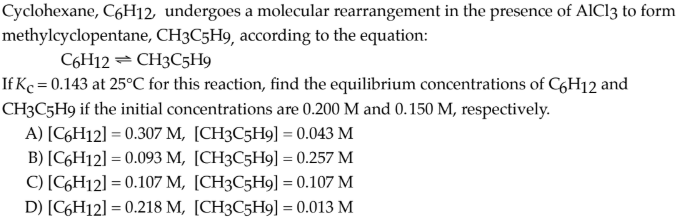# Cyclohexane, C6H12, undergoes a molecular rearrangement in the presence of AlCl3 to form methylcyclopentane, CH3C5H9, according to the equation: C6H12 ⇌ CH3C5H9 If Kc = 0.143 at 25°C for this reaction, find the equilibrium concentrations of C6H12 and CH3C5H9 if the initial concentrations are 0.200 M and 0.150 M, respectively. A) [C6H12] = 0.307 M, [CH3C5H9] = 0.043 M B) [C6H12] = 0.093 M, [CH3C5H9] = 0.257 M C) [C6H12] = 0.107 M, [CH3C5H9] = 0.107 M D) [C6H12] = 0.218 M, [CH3C5H9] = 0.013 M